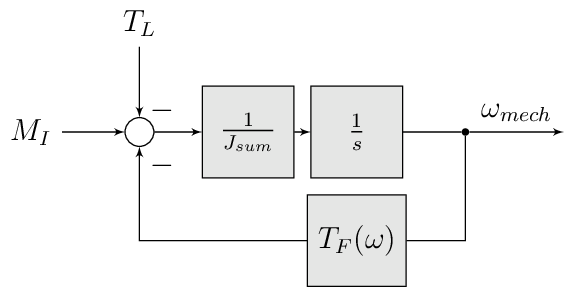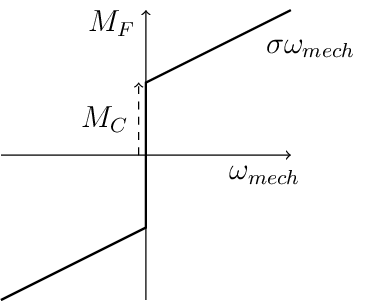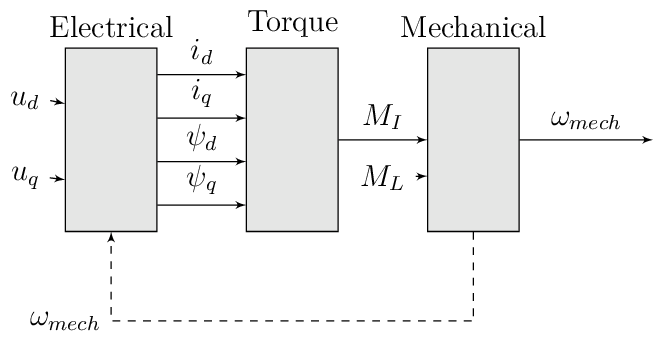# PMSM Model

• IP-Core of a PMSM model

• Simulates a PMSM on the FPGA

• Intended for controller-in-the-loop (CIL) on the UltraZohm

• Time discrete transformation is done by zero order hold transformation

• Sample frequency of the integrator is $$T_s=\frac{1}{2\,MHz}$$

• IP-Core clock frequency must be $$f_{clk}=100\,MHz$$!

• IP-Core has single precision AXI ports

• All calculations in the IP-Core are done in double precision!

## System description

### Electrical System

The model assumes a symmetric machine with a sinusoidal input voltage as well as the common assumptions for the dq-transformation (neglecting the zero-component). Small letter values indicate time dependency without explicitly stating it. The PMSM model is based on its differential equation using the flux-linkage as state values in the dq-plane [2, p. 1092]:

\begin{align}\begin{aligned}\frac{d \psi_d}{dt} &= u_d - R_1 i_d + \omega_{el} \psi_q\\\frac{d \psi_q}{dt} &= u_q - R_1 i_q - \omega_{el} \psi_d\end{aligned}\end{align}

The flux-linkages of the direct and quadrature axis are given by [2, p. 1092]:

\begin{align}\begin{aligned}\psi_d &= \psi_{pm} + L_d i_d\\\psi_q &= L_q i_q\end{aligned}\end{align}

Rearranging to calculate the current from the flux-linkage:

\begin{align}\begin{aligned}i_d &= \frac{\psi_d - \psi_{pm}}{L_d}\\i_q &= \frac{\psi_q}{L_q}\end{aligned}\end{align}

With the rotational speed linked to the electrical rotation speed in dq-coordinates by the number of pole pairs [2, p. 1092]:

$\omega_{el}=p \cdot \omega_{mech}$

The PMSM generates an inner torque $$T_I$$ according to:

$T_I=\frac{3}{2}p(\psi_d i_q - \psi_q i_d)$

This can be rearranged to the following equation [2, p. 1092]. Note that the flux-based equation above is implemented in the model.

$T_I=\frac{3}{2} p \big(i_q \psi_{pm} + i_d i_q (L_d -L_q) \big)$

### Mechanical system

The mechanical system is modeled by the following equations. The inertia of the complete system is summed into the inertia $$J_{sum}$$, i.e., rigid coupling of the system is assumed.

$\frac{d \omega_{mech}}{dt} = \frac{ T_I - T_F - T_L }{J_{sum}}$Fig. 129 Block diagram of mechanical system

#### Friction

The friction $$M_F(\omega)$$ [ 1, p. 12 ff] is implemented with the simplified viscous friction model:

$M_F = sign(\omega_{mech}) \cdot M_c + \sigma \omega_{mech}$

With the constant coulomb friction $$M_c$$, and the friction coefficient $$\sigma$$.Fig. 130 Friction model [ 1, p. 13]

## IP-Core overviewFig. 131 Block diagram of IP-Core

All time-dependent variables are either inputs or outputs that are written/read by AXI4-full. That is, $$u_d$$, $$u_q$$, $$\omega_{mech}$$, and $$M_L$$ are inputs. Furthermore, $$i_d$$, $$i_q$$, $$M_I$$, and $$\omega_{mech}$$ are outputs. The IP-Core inputs $$\boldsymbol{u}(k)=[{v}_{d} ~ v_{q} ~ T_{L}]$$ and outputs $$\boldsymbol{y}(k)=[i_{d} ~ i_{q} ~ T_{L} ~ \omega_{m}]$$ are accessible by AXI4 (including burst transactions). Furthermore, all machine parameters, e.g., stator resistance, can be written by AXI at runtime. All AXI-transactions use single-precision variables, which the IP-Core converts to and from double precision. The inputs $$\boldsymbol{u}(k)$$ and outputs $$\boldsymbol{y}(k)$$ use a shadow register that holds the value of the register until a sample signal is triggered. Upon triggering, the inputs from the shadow register are passed to the actual input registers of the IP-Core, and the current output $$\boldsymbol{y}(k)$$ is stored in the output shadow register (strobe functions of driver). The shadow registers can be triggered according to the requirements of the controller in the loop and ensure synchronous read/write operations. The inputs and outputs are implemented as an vector, therefore the HDL-Coder adds the strobe / shadow register automatically - it is not visible in the model itself. Note that $$\omega_{mech}$$ is an input as well as an output. The IP-Core has two modes regarding the rotational speed $$\omega_{mech}$$:

1. Simulate the mechanical system and calcualte $$\omega_{mech}$$ according to the equations in Friction.

2. Use the rotational frequency $$\omega_{mech}$$ that is written as an input (written by AXI).

When the flag simulate_mechanical_system is true, the rotational speed in the output struct is calculated by the IP-Core, and the input value of the rotational speed has no effect. When the flag simulate_mechanical_system is false, the rotational speed in the output struct is equal to the rotational speed of the input. This behavior is implemented in the hardware of the IP-Core with switches. The input and output values are intended to be written and read in a periodical function, e.g., the ISR.

In addition to the time-dependent values, the PMSM model parameters are configured by AXI.

### Integration

The differential equations of the electrical and mechanical system are discretized using the explicit Euler method [ 3, p. 3 ]. Using this method is justified by the small integration step of the implementation ($$t_s=0.5~\mu s$$) and is a commonly used approach 3, p. 3 ]. The new value at time $$k+1$$ of the state variable is calcualted for every time step based on the old values ($$k$$):

\begin{align}\begin{aligned}\psi_d(k+1) &= t_s \bigg( u_d(k) - R_1 i_d(k) + \omega_{el} \psi_q(k) \bigg) + \psi_d(k)\\\psi_q(k+1) &=t_s \bigg( u_q(k) - R_1 i_q(k) - \omega_{el} \psi_d(k) \bigg) + \psi_q(k)\end{aligned}\end{align}

For the mechanical system:

$\omega_{mech}(k+1) =ts \bigg( \frac{ T_I(k) - T_F(k) - T_L(k) }{J_{sum}} \bigg) + \omega_{mech}(k)$

### IP-Core Hardware

• The module takes all inputs and converts them from single precision to double precision.

• The output is converted from double precision to single precision (using rounding to the nearest value in both cases).

• All input values are adjustable at run-time

• The sample time is fixed!

• The IP-Core uses Native Floating Point of the HDL-Coder

• Several parameters are written as their reciprocal to the AXI register to make the calculations on hardware simple (handled by the driver!)

• The IP-Core uses an oversampling factor of 50

• Floating Point latency Strategy is set to MIN

• Handle denormals is activated

## Example usage

• Source IPCORE_CLK with a $$100\,MHz$$ clock!

• Connect other ports accordingly

• Build bitstream, export .xsa, update Vitis platform

### Vitis

• Initialize the driver in main and couple the base address with the driver instance

Listing 164 Changes in main.c (R5)
#include "IP_Cores/uz_pmsmMmodel/uz_pmsmModel.h"
#include "xparameters.h"
uz_pmsmModel_t *pmsm=NULL;

int main(void) {
// other code...

struct uz_pmsmModel_config_t pmsm_config={
.ip_core_frequency_Hz=100000000,
.simulate_mechanical_system = true,
.r_1 = 2.1f,
.L_d = 0.03f,
.L_q = 0.05f,
.psi_pm = 0.05f,
.polepairs = 2.0f,
.inertia = 0.001,
.coulomb_friction_constant = 0.01f,
.friction_coefficient = 0.001f};

pmsm=uz_pmsmModel_init(pmsm_config);
// before ISR Init!
// more code of main

• Read and write the inputs in isr.c

• Add before ISR with global scope to use the driver and Waveform Generator:

Listing 165 Changes in isr.c
#include "../uz/uz_wavegen/uz_wavegen.h"
#include "../IP_Cores/uz_pmsmMmodel/uz_pmsmModel.h"
extern uz_pmsmModel_t *pmsm;

float i_d_soll=0.0f;
float i_q_soll=0.0f;
struct uz_pmsmModel_inputs_t pmsm_inputs={
.omega_mech_1_s=0.0f,
.v_d_V=0.0f,
.v_q_V=0.0f,
};

struct uz_pmsmModel_outputs_t pmsm_outputs={
.i_d_A=0.0f,
.i_q_A=0.0f,
.torque_Nm=0.0f,
.omega_mech_1_s=0.0f
};

void ISR_Control(void *data){
// other code
uz_pmsmModel_trigger_input_strobe(pmsm);
uz_pmsmModel_trigger_output_strobe(pmsm);
pmsm_outputs=uz_pmsmModel_get_outputs(pmsm);
pmsm_inputs.v_q_V=uz_wavegen_pulse(10.0f, 0.10f, 0.5f);
pmsm_inputs.v_d_V=-pmsm_inputs.v_q_V;
uz_pmsmModel_set_inputs(pmsm, pmsm_inputs);
// [...]
}

• Change the Javascope enum to transfer the required measurement data

Listing 166 Adjust JS_OberservableData enum in javascope.h (R5) to measure pmsm_outputs
// Do not change the first (zero) and last (end) entries.
enum JS_OberservableData {
JSO_ZEROVALUE=0,
JSO_i_q,
JSO_i_d,
JSO_omega,
JSO_v_d,
JSO_ENDMARKER
};

• Configure the Javascope to transmit the pmsm output data:

Listing 167 Adjust JavaScope_initalize function in javascope.c (R5) to measure pmsm_outputs
  #include "../IP_Cores/uz_pmsmMmodel/uz_pmsmModel.h"
extern struct uz_pmsmModel_outputs_t pmsm_outputs;
extern struct uz_pmsmModel_inputs_t pmsm_inputs;

int JavaScope_initalize(DS_Data* data){
// existing code
// [...]
// Store every observable signal into the Pointer-Array.
// With the JavaScope, 4 signals can be displayed simultaneously
// Changing between the observable signals is possible at runtime in the JavaScope.
// the addresses in Global_Data do not change during runtime, this can be done in the init
js_ch_observable[JSO_i_q] = &pmsm_outputs.i_q_A;
js_ch_observable[JSO_i_d] = &pmsm_outputs.i_d_A;
js_ch_observable[JSO_omega] = &pmsm_outputs.omega_mech_1_s;
js_ch_observable[JSO_v_d] = &pmsm_inputs.v_d_V;
return Status;
}


### Javascope

• Make sure that in properties.ini, smallestTimeStepUSEC = 50 is set

### Comparison between reference and IP-Core

• Program UltraZohm with included PMSM IP-Core and software as described above

• Start Javascope

• Connect to javascope, set scope to running and time scale to 100x

• Start logging of data after a falling edge on the setpoint and stop at the next fallning edge

• Copy measured .csv data to ultrazohm_sw/ip-cores/uz_pmsm_model

• Rename it to open_loop_mearuement.csv

• Run compare_simulation_to_measurement.m in ultrazohm_sw/ip-cores/uz_pmsm_modelFig. 138 Comparison of step response between the reference model and IP-Core implementation measured by Javascope

### Closed loop

uz_pmsmModel_trigger_input_strobe(pmsm);
uz_pmsmModel_trigger_output_strobe(pmsm);
pmsm_outputs=uz_pmsmModel_get_outputs(pmsm);
referenceValue=uz_wavegen_pulse(1.0f, 0.10f, 0.5f);
pmsm_inputs.v_q_V=uz_PI_Controller_sample(pi_q, referenceValue, pmsm_outputs_old.i_q_A, false);
pmsm_inputs.v_d_V=uz_PI_Controller_sample(pi_d, -referenceValue, pmsm_outputs_old.i_d_A, false);
pmsm_inputs.v_q_V+=pmsm_config.polepairs*pmsm_outputs_old.omega_mech_1_s*(pmsm_config.L_d*pmsm_outputs_old.i_d_A+pmsm_config.psi_pm);
pmsm_inputs.v_d_V-=pmsm_config.polepairs*pmsm_outputs_old.omega_mech_1_s*(pmsm_config.L_q*pmsm_outputs_old.i_q_A);
uz_pmsmModel_set_inputs(pmsm, pmsm_inputs);
pmsm_outputs_old=pmsm_outputs;


## Driver reference

typedef struct uz_pmsmModel_t uz_pmsmModel_t

Object data type definition of the PMSM model IP-Core driver.

struct uz_pmsmModel_config_t

Configuration struct for the PMSM model IP-Core driver.

Public Members

Base address of the IP-Core instance to which the driver is coupled

uint32_t ip_core_frequency_Hz

Clock frequency of IP-Core

float polepairs

Pole pairs of the PMSM

float r_1

Stator resistance in ohm

float L_d

Direct inductance in Henry

float L_q

float psi_pm

Linked magnetic flux of PM magnets

float friction_coefficient

Linear coefficient of friction

float coulomb_friction_constant

Static friction constant

float inertia

Inertia of the PMSM

bool simulate_mechanical_system

Determine if mechanical system is simulated or speed is an input

struct uz_pmsmModel_outputs_t

Struct to return and read the outputs of the PMSM Model.

Public Members

float i_d_A

Current in d-axis in A

float i_q_A

Current in q-Axis in A

float torque_Nm

Inner torque of PMSM in Nm

float omega_mech_1_s

Rotational speed of PMSM in 1/s

struct uz_pmsmModel_inputs_t

Struct to be used to pass inputs to the PMSM Model.

Public Members

float v_d_V

Voltage in d-axis in V

float v_q_V

Voltage in q-axis in V

float omega_mech_1_s

Rotational speed of PMSM in 1/s

uz_pmsmModel_t *uz_pmsmModel_init(struct uz_pmsmModel_config_t config)

Initialize an instance of the driver.

Parameters
• config – Config struct

Returns

uz_pmsmModel_t* Pointer to an initialized instance of the driver

void uz_pmsmModel_set_inputs(uz_pmsmModel_t *self, struct uz_pmsmModel_inputs_t inputs)

Set inputs of the model and write them to the PMSM model IP-Core.

Parameters
• self – Pointer to driver instance

• inputs – Inputs to be written to IP-Core

struct uz_pmsmModel_outputs_t uz_pmsmModel_get_outputs(uz_pmsmModel_t *self)

Returns current outputs of PMSM model IP-Core.

Parameters
• self – Pointer to driver instance

Returns

struct uz_pmsmModel_outputs_t Output values

void uz_pmsmModel_reset(uz_pmsmModel_t *self)

Resets the PMSM model by writing zero to all inputs and sets integrators to zero.

Parameters
• self – Pointer to driver instance

void uz_pmsmModel_trigger_input_strobe(uz_pmsmModel_t *self)

Takes the values of the AXI shadow register and pass them to the actual input.

Parameters
• self

void uz_pmsmModel_trigger_output_strobe(uz_pmsmModel_t *self)

Takes the values of the shadow register and pass them to the actual AXI register.

Parameters
• self

### Sources

1(1,2)

Zur Modellierung und Kompensationdynamischer Reibung in Aktuatorsystemen, Michael Ruderman, Dissertation, 2012, TU Dortmund (German)

2(1,2,3,4)

Elektrische Antriebe - Regelung von Antriebssystemen, Dierk Schröder, Springer, 2015, 4. Edition (German)

3(1,2)

Exploring the Limits of Floating-Point Resolution for Hardware-In-the-Loop Implemented with FPGAs, Alberto Sanchez, Elías Todorovich, and Angel De Castro, Applications of Power Electronics, https://doi.org/10.3390/electronics7100219